## 3.系统硬件设计

### 3.1 电源管理模块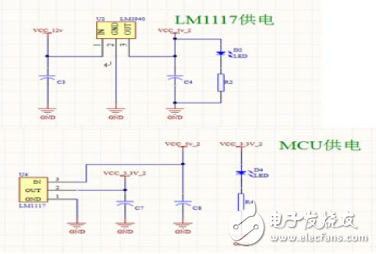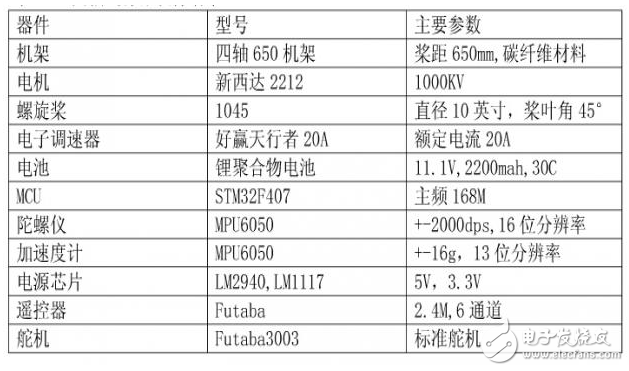## 4.系统软件设计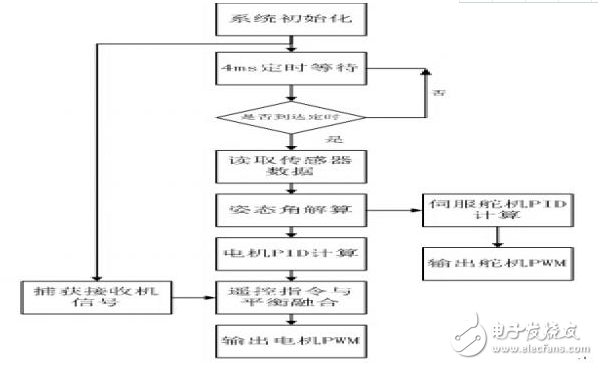4.1.四元素计算姿态角的实现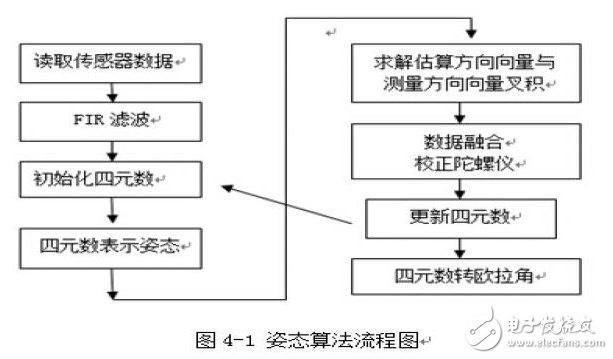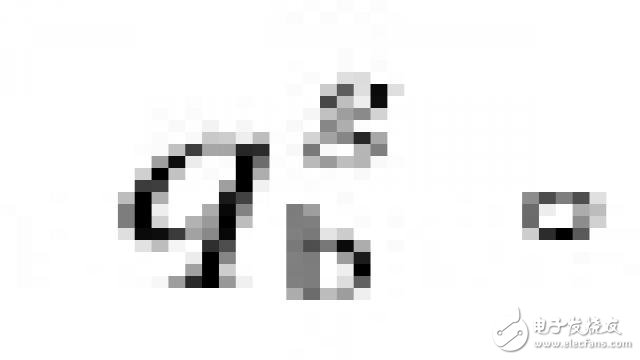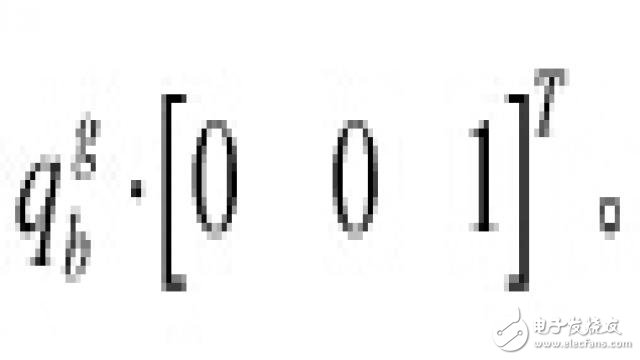。所以加速的向量与估计重力向量叉积：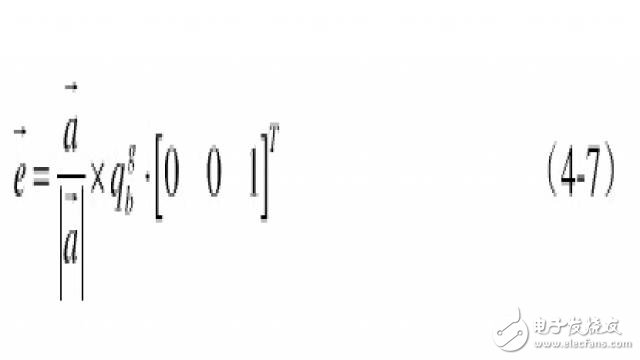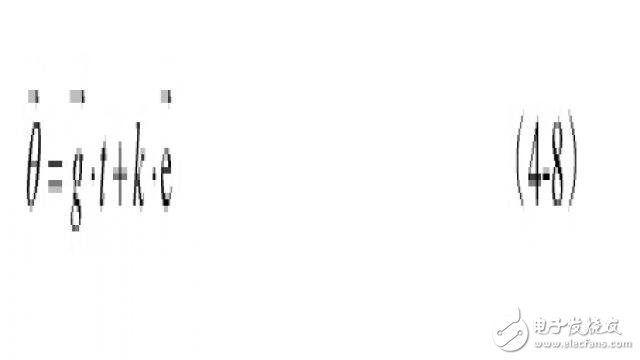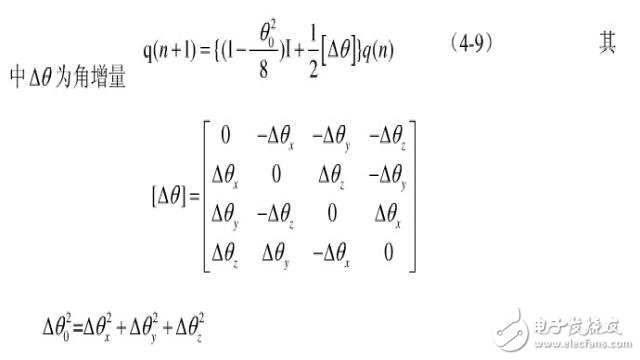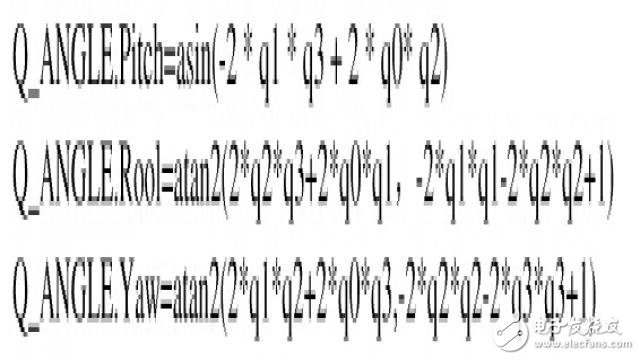### 4.2.控制设计#define PIDMIX(X,Y,Z) Motor_Thr + pid_pitch* Y+ pid_roll*X + pid_yaw*Z

MOTOR1=MOTORLimit(PIDMIX(+1,+1,-1));       //REAR_R  后右电机

MOTOR2=MOTORLimit(PIDMIX(-1,-1,-1));           //FRONT_R 前右电机

MOTOR3=MOTORLimit(PIDMIX(-1,+1,+1));         //REAR_L  后左电机

MOTOR4=MOTORLimit(PIDMIX(+1,-1,+1));         //FRONT_L 前左电机

### 4.3. PID参数调节

PID参数的整定是PID控制的关键环节，直接影响到控制的效果。故一个PID设计的好不好往往要看其参数能否调节好，本设计的PID参数调节采用凑试法。凑试法是通过实际的闭环系统，通过观察系统的响应曲线，在本设计中通过观察被调量，PID输出，设定值三条曲线，判断出kp,ki,kd对系统响应的影响，反复尝试，最终达到满意响应，从而达到确定控制参数的kp,ki,kd的目的。在参数调节过程总遵循以下原则:

（1）在输出不振荡时，增大比例增益P。

（2）在输出不振荡时，减小积分时间常数TI。

（3）在输出不振荡时，增大微分时间常数Td。

（4）一般步骤：

a.确定比例增益P

b.确定积分时间常数TI

c.确定积分时间常数Td

d.系统空载、带载联调，再对PID参数进行微调，直至满足要求。

（5）最终调试好的标准应该是，PID输出曲线在有一个阶跃响应来是，响应一大一小两个波，小波是大波的四分之一。

## 6.评测与结论

### 6.1.四轴飞行器的调试

6.1.1电源调试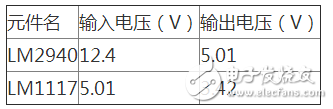6.1.2 姿态角调试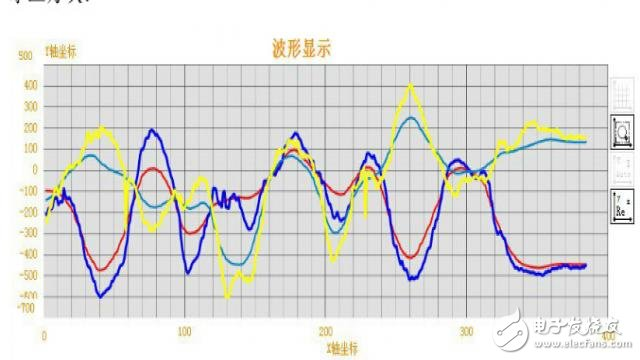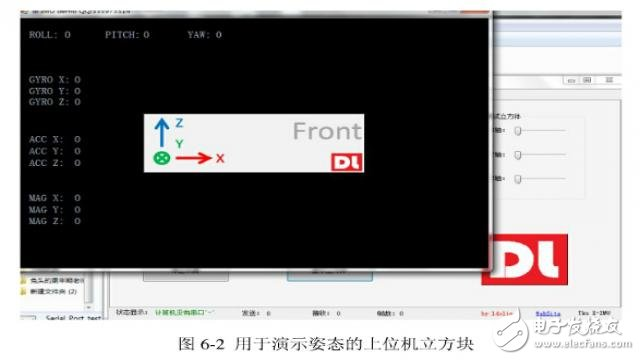6.1.3 控制电机调试

### 6.2.四轴飞行器的试飞

void IMUupdate(float gx, float gy, float gz, float ax, float ay, float az)

{

static float q0 = 1.0f, q1 = 0.0f, q2 = 0.0f, q3 = 0.0f;

float delta_2=0;

const static float FACTOR = 0.002;

float norm=0.0f;

float vx, vy, vz;

float ex, ey, ez;

float q0q0 = q0*q0;

float q0q1 = q0*q1;

float q0q2 = q0*q2;

float q1q1 = q1*q1;

float q1q3 = q1*q3;

float q2q2 = q2*q2;

float q2q3 = q2*q3;

float q3q3 = q3*q3;

norm = sqrt(ax*ax + ay*ay +az*az);       // 测量正常化 把加速度计的三维向量转成单位向量。

ax = ax /norm;

ay = ay / norm;

az = az / norm;

vx = 2*(q1q3 - q0q2);                                         // 估计方向的重力

vy = 2*(q0q1 + q2q3);

vz = q0q0 - q1q1 - q2q2 + q3q3;

ex = (ay*vz - az*vy);

ey = (az*vx - ax*vz);

ez = (ax*vy - ay*vx);

halfT=0.002;

gx = gx + ex*FACTOR/halfT;                                 //校正陀螺仪测量值    用叉积误差来做PI修正陀螺零偏

gy = gy + ey*FACTOR/halfT;

gz = gz + ez*FACTOR/halfT;

delta_2=(2*halfT*gx)*(2*halfT*gx)+(2*halfT*gy)*(2*halfT*gy)+(2*halfT*gz)*(2*halfT*gz);

q0 = (1-delta_2/8)*q0 + (-q1*gx - q2*gy - q3*gz)*halfT;                    // 整合四元数率       四元数微分方程       四元数更新算法，二阶毕卡法

q1 = (1-delta_2/8)*q1 + (q0*gx + q2*gz - q3*gy)*halfT;

q2 = (1-delta_2/8)*q2 + (q0*gy - q1*gz + q3*gx)*halfT;

q3 = (1-delta_2/8)*q3 + (q0*gz + q1*gy - q2*gx)*halfT;

norm = sqrt(q0*q0 + q1*q1 + q2*q2 + q3*q3);        // 正常化四元

q0 = q0 / norm;

q1 = q1 / norm;

q2 = q2 / norm;

q3 = q3 / norm;

//转换为欧拉角

Q_ANGLE.Pitch  = asin(-2 * q1 * q3 + 2 * q0* q2)* 57.3;                                   // pitch

Q_ANGLE.Roll = atan2(2 * q2 * q3 + 2 * q0 * q1, -2 * q1 * q1 - 2 * q2* q2 + 1)* 57.3;    // roll

Q_ANGLE.Yaw = -atan2(2 * q1 * q2 + 2 * q0 * q3, -2 * q2*q2 - 2 * q3 * q3 + 1)* 57.3; // yaw

}

STM32/STM8

• 没有资料